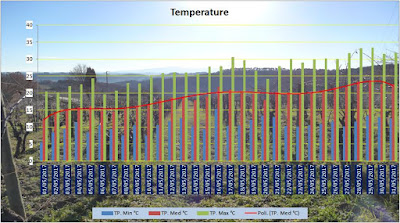## Posts

Showing posts from June, 2017

### 2017 May weatherDaily temperature Max-Med-Min [°C] highest temp= 33,4 °C  lowest temp= 5,5 °C mean temp=18,7°C mean of the max=26,7°C mean of the min=10,7 °C Max temperature difference =  21,6 °C Mean temperature difference = 16°C Daily rain [mm] Mean rain=0,34mm Total rain= 10,8 mm Daily umidity Max - Med - Min Mean umidity=64% Mean of the min=35% Mean Solar Radiation [W/m2] Mean value=275 W/m2

### 2017 April weather20, 21 and 22 night vines were frosted Daily temperature Max-Med-Min [°C] highest temp= 26,7 °C  lowest temp= -1 °C mean temp=14,0°C mean of the max=22,3°C mean of the min=6,6 °C Max temperature difference =  20,3 °C Mean temperature difference = 15,2°C Daily rain [mm] Mean rain=0,45mm Total rain=13,6mm Daily umidity Max - Med - Min Mean umidity=68% Mean of the min=36% Mean Solar Radiation [W/m2] Mean value=172 W/m2